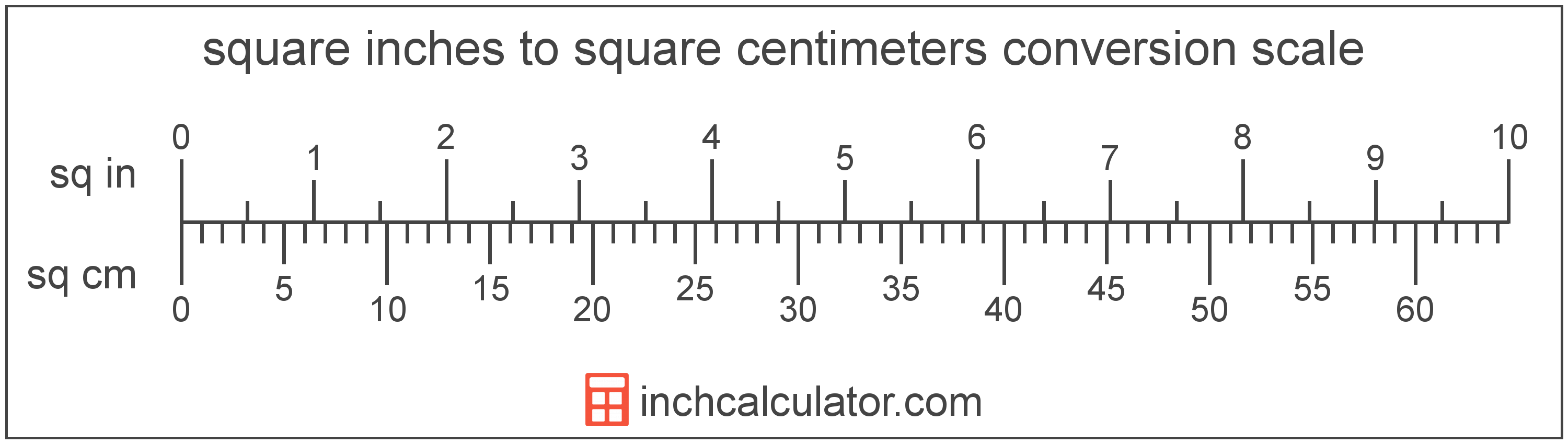# Square Inches to Square Centimeters Conversion

Enter the area in square inches below to get the value converted to square centimeters.

Results in Square Centimeters:1 sq in = 6.4516 sq cm

## How to Convert Square Inches to Square Centimeters

To convert a square inch measurement to a square centimeter measurement, multiply the area by the conversion ratio.

Since one square inch is equal to 6.4516 square centimeters, you can use this simple formula to convert:

square centimeters = square inches × 6.4516

The area in square centimeters is equal to the square inches multiplied by 6.4516.

For example, here's how to convert 5 square inches to square centimeters using the formula above.
5 sq in = (5 × 6.4516) = 32.258 sq cm## Square Inches

One square inch is equal to the area of a square with sides that are 1 inch in length.

The square inch is a US customary and imperial unit of area. A square inch is sometimes also referred to as a square in. Square inches can be abbreviated as sq in, and are also sometimes abbreviated as in². For example, 1 square inch can be written as 1 sq in or 1 in².

## Square Centimeters

One square centimeter is equal to the area of a square with 1 centimeter long sides.

The square centimeter, or square centimetre, is a multiple of the square meter, which is the SI derived unit for area. In the metric system, "centi" is the prefix for 10-2. A square centimeter is sometimes also referred to as a square cm. Square centimeters can be abbreviated as sq cm, and are also sometimes abbreviated as cm². For example, 1 square centimeter can be written as 1 sq cm or 1 cm².

## Square Inch to Square Centimeter Conversion Table

Square inch measurements converted to square centimeters
Square Inches Square Centimeters
1 sq in 6.4516 sq cm
2 sq in 12.9 sq cm
3 sq in 19.35 sq cm
4 sq in 25.81 sq cm
5 sq in 32.26 sq cm
6 sq in 38.71 sq cm
7 sq in 45.16 sq cm
8 sq in 51.61 sq cm
9 sq in 58.06 sq cm
10 sq in 64.52 sq cm
11 sq in 70.97 sq cm
12 sq in 77.42 sq cm
13 sq in 83.87 sq cm
14 sq in 90.32 sq cm
15 sq in 96.77 sq cm
16 sq in 103.23 sq cm
17 sq in 109.68 sq cm
18 sq in 116.13 sq cm
19 sq in 122.58 sq cm
20 sq in 129.03 sq cm
21 sq in 135.48 sq cm
22 sq in 141.94 sq cm
23 sq in 148.39 sq cm
24 sq in 154.84 sq cm
25 sq in 161.29 sq cm
26 sq in 167.74 sq cm
27 sq in 174.19 sq cm
28 sq in 180.64 sq cm
29 sq in 187.1 sq cm
30 sq in 193.55 sq cm
31 sq in 200 sq cm
32 sq in 206.45 sq cm
33 sq in 212.9 sq cm
34 sq in 219.35 sq cm
35 sq in 225.81 sq cm
36 sq in 232.26 sq cm
37 sq in 238.71 sq cm
38 sq in 245.16 sq cm
39 sq in 251.61 sq cm
40 sq in 258.06 sq cm